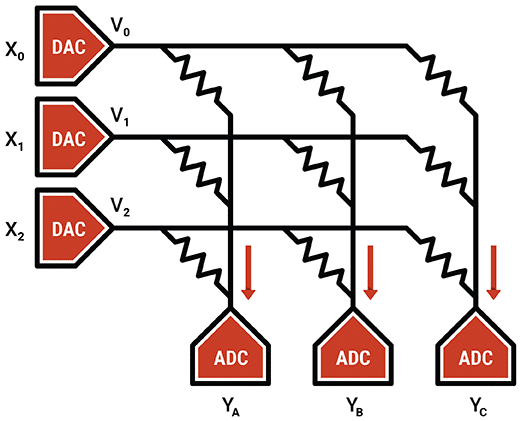# Analog Computing

### Achieving unmatched efficiency and performanceAnalog computing provides the ultimate compute-in-memory processing element. The term compute-in-memory is used very broadly and can mean many things. Our analog compute takes compute-in-memory to an extreme, where we compute directly inside the memory array itself. This is possible by using the memory elements as tunable resistors, supplying the inputs as voltages, and collecting the outputs as currents. We use analog computing for our core neural network matrix operations, where we are multiplying an input vector by a weight matrix.

Analog computing provides several key advantages. First, it is amazingly efficient; it eliminates memory movement for the neural network weights since they are used in place as resistors. Second, it is high performance; there are hundreds of thousands of multiply-accumulate operations occurring in parallel when we perform one of these vector operations. Given these two properties, analog computing is the core of our high-performance yet highly-efficient system.